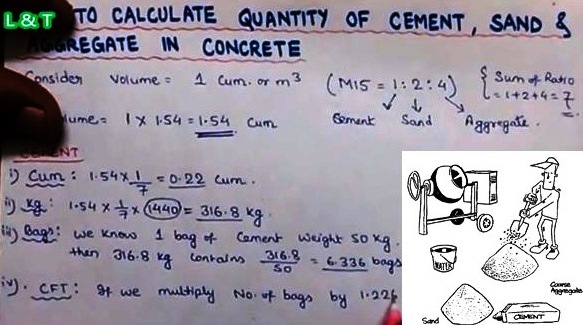Estimating Cement, Sand and Aggregate Quantity in Concrete

# Estimating Cement, Sand and Aggregate Quantity in ConcreteQuantities of materials for the production of required quantity of concrete of given mix proportions can be calculated by absolute volume method. This method is based on the principle that the volume of fully compacted concrete is equal to the absolute volume of all the materials of concrete, i.e. cement, sand, coarse aggregates and water.
A Concrete structure may consists of beams, slabs, columns and foundations etc. based on type of structure. The volume of concrete required for concrete structure can be calculated by summing up the volumes of each structural member or each parts of members. The volume of a rectangular cross sectional member can be calculated as length x width x height (or depth or thickness). Suitable formula shall be used for different cross-sectional shapes of members.
The formula for calculation of materials for required volume of concrete is given by:Where, Vc = Absolute volume of fully compacted fresh concrete
W =Mass of water
C = Mass of cement
Fa = Mass of fine aggregates
Ca = Mass of coarse aggregates
Sc, Sfa and Sca are the specific gravities of cement, fine aggregates and coarse aggregates respectively.
The air content has been ignored in this calculation.
This construction video provides detailed methods for estimating the quantity of cement, sand and aggregate in cement concrete to figure out the cost of all the materials. In this video, the volume of PCC to be prepared is taken as 10 cubic meter that is to be completed with M15 grade of concrete in the ratio of 1:2:4.
This video has nicely demonstrated about the detailed calculation step by step Credits on this

Continue Reading about the "Estimating Cement, Sand and Aggregate Quantity in Concrete" on the next page below

1.2.